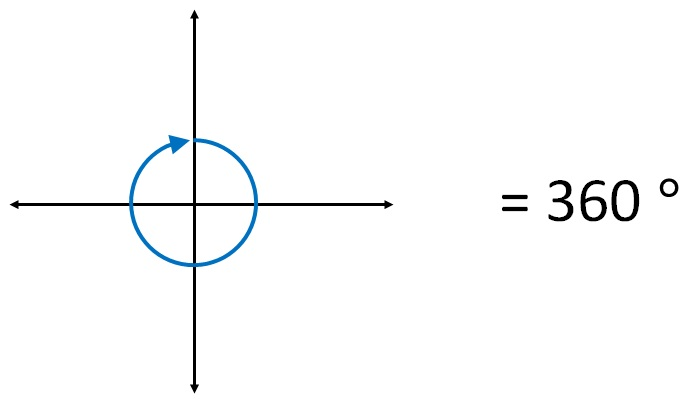Measuring angles

Chapter 5 Class 6 Understanding Elementary Shapes
Concept wise1 Full revolution = 360 °

Degree

We measure angles in term of degrees.

1 revolution = 360 °

1/2 revolution = (360°)/2 = 180°

1/4 revolution = (360°)/4 = 90°

So,

Right angle =  1/4 revolution = 90°

Straight angle = 1/2 revolution = 180°

Acute Angle

Acute angle is less that right angle.

∴        Acute angle < Right Angle.

Acute angle < 90°

Obtuse Angle

Obtuse angle is greater than right angle, but smaller than straight angle.

Right angle < Obtuse angle < Straight angle.

90° < Obtuse angle < 180°

Reflex Angle

Reflex angle is an angle greater than straight angle.

Reflex angle > Straight angle

Reflex angle > 180°

To summarize,

Right angle = 90°

Straight = 180°

Acute angle < 90°

Obtuse angle > 90° & Obtuse angle < 180°

Reflex angle > 180°

Learn in your speed, with individual attention - Teachoo Maths 1-on-1 Class